# C Programming Examples

Programming is like mathematics. As in mathematics, theory is not enough. To clearly understand the topic, you must practice solving the problems related to it. Just like this, in programming, to learn any topic, you must practice coding (with yourself) related to the topic.

So here we have listed more than 200 programs with their output and a step-by-step explanation. Everything from the most basic C programs to shutting down your computer with a C program can be found here.

But before going through all those programs, let's first take a look at some of the interesting ones given here. I'm sure that, after having a look at the output of these programs listed here, your interest in programming will increase. Don't freak out when you see the code; you have no idea what's going on inside. You will learn more than you think about C programming over here.

The step-by-step explanation of all the programs starts on the next page. However, these programs are only a sample; you will learn much more about them on the following page.

I'm sure that, after learning all the programs (starts on the next page), you will be able to understand the programs given below and can also modify them yourself. And maybe you can create some programs that are beyond my thinking.

And I'm also sure you can create a lot of programs that are not listed here. But before going to the demo programs listed in this article, let's have a look at some popular programs available in the C language:

## C Examples - Demo Programs

As I've already said at the beginning of this article, after having a look at the output of these programs, you will get more interested in learning C. Let's get started with one of the simplest programs available in C.

### C Program Example No. 1

The following is the simplest C program that will print "Hello Compiler, I am C" on the screen :

```#include<stdio.h>
#include<conio.h>
int main()
{
printf("Hello Compiler, I am C");
getch();        // holds the output screen until the user press a key
return 0;
}```

This program was built and runs under the Code::Blocks IDE. Here is the output produced by this program: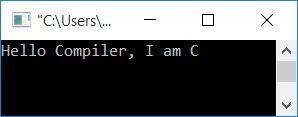### C Program Example No. 2

Here is the second program, where the user is allowed to enter any input.

```#include<stdio.h>
#include<conio.h>
int main()
{
char name;
scanf("%s", name);
printf("Hello, %s\nWelcome to codescracker.com", name);
getch();
return 0;
}```

Here is the sample run of the previous (above) program:### C Program Example No. 3

This is a password-protected program. That is, the user is allowed to create a password and then enter it to see the result.

```#include<stdio.h>
#include<conio.h>
#include<string.h>
int main()
{
char pass, e_pass;
int num1, num2, sum;
scanf("%s", pass);
printf("\nEnter any two number to Add: ");
scanf("%d %d", &num1, &num2);
printf("\nEnter password to see the Result: ");
scanf("%s", e_pass);
if(!strcmp(pass, e_pass))
{
sum = num1 + num2;
printf("\n%d + %d = %d", num1, num2, sum);
}
else
printf("\nSorry! You entered a Wrong Password");
getch();
return 0;
}```

The sample run of the above C program in both cases (if the user enters the correct or wrong password) is shown here. This is the first output if the user enters the wrong password:If the user enters the correct password, this is the second one: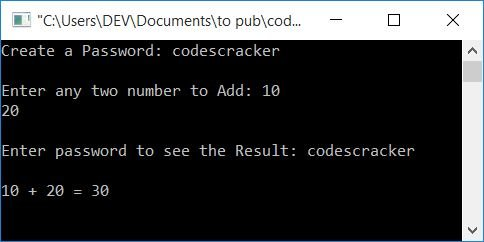### C Program Example No. 4

This program receives a string input from the user and compares the string with yes to print the message according to the input. Don't worry, you'll learn everything one by one, beginning with the next page.

```#include<stdio.h>
#include<conio.h>
#include<string.h>
int main()
{
char str;
printf("Are you a Programmer ? ");
scanf("%s", str);
if(!strcmp("yes", str))
printf("\nWhat a True Mind, You have!");
else
printf("\nSorry! You are telling a lie.");
getch();
return 0;
}```

This is the first output: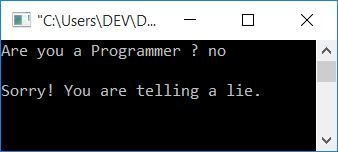If you are here, then you are interested in learning programming. So, assuming you're a programmer, why did we enter "no"?
Let's try to go for "yes". The following is the second output: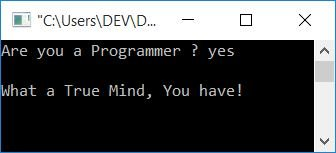### C Program Example No. 5

Here is another program in C that checks for invited members and prints whether the current user is invited or not based on user input.

```#include<stdio.h>
#include<conio.h>
#include<string.h>
int main()
{
char arr = {"programmer", "developer", "engineer",
"student", "teacher", "professor"};
char name;
int found=0;
printf("Who are You ? ");
scanf("%s", name);
for(int i=0; i<6; i++)
{
if(!strcmp(name, arr[i]))
{
printf("\nCongratulation!!");
printf("\nYou're invited from codescracker.com.");
found=1;
break;
}
else
found++;
}
if(found != 1)
printf("\nSorry!\nYou're not invited.");
getch();
return 0;
}```

The sample run of the above C program in both cases is shown here. The following is the first output: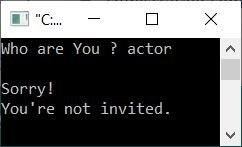The second is as follows: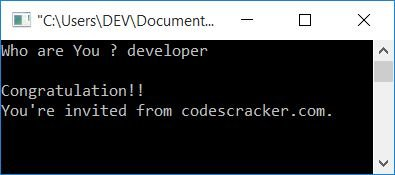### C Program Example No. 6

This is the last demo program. That is, on the next page, you will see programs with their output and a step-by-step explanation of the code.

```#include<stdio.h>
#include<conio.h>
int main()
{
int starTot, rowTot, i, j, starInOneRow, rem;
printf("How many stars you want to print ? ");
scanf("%d", &starTot);
printf("Upto how many rows ? ");
scanf("%d", &rowTot);
starInOneRow = starTot/rowTot;
rem = starTot%rowTot;
printf("\n");
i=0;
while(i<rowTot)
{
if(i!=0)
printf("\n");
for(j=0; j<starInOneRow; j++)
printf("* ");
i++;
}
if(rem!=0)
{
for(i=0; i<rem; i++)
printf("* ");
}
getch();
return 0;
}```

The snapshot given below is its sample run: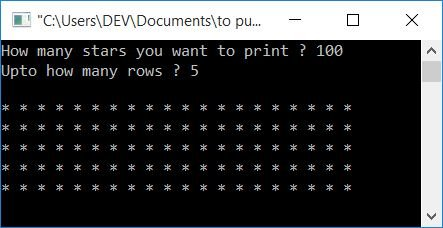This is the second sample run:From here on out, you will be introduced to the world of C programming, from the fundamentals to the advanced level. A step-by-step explanation of all the programs is given.

C Quiz

« C Tutorial Next Program »